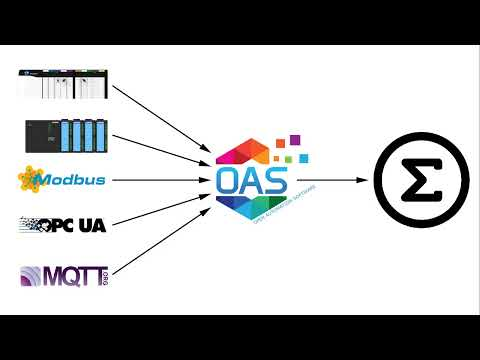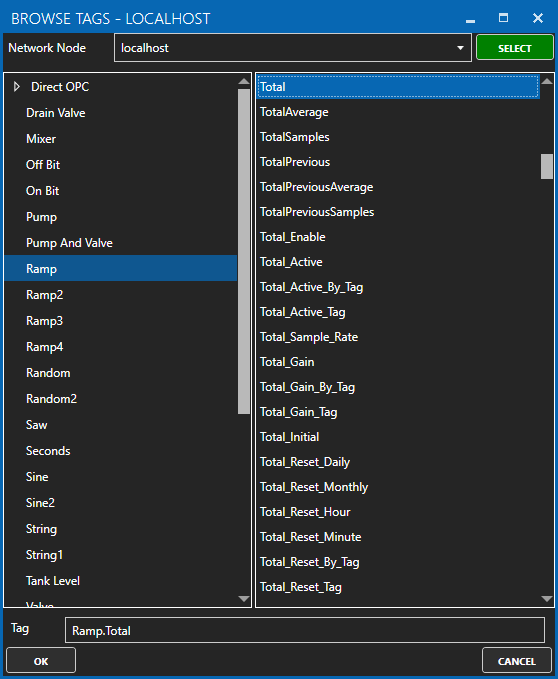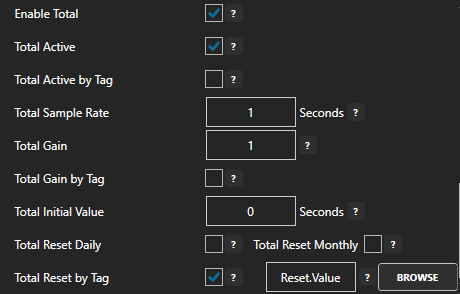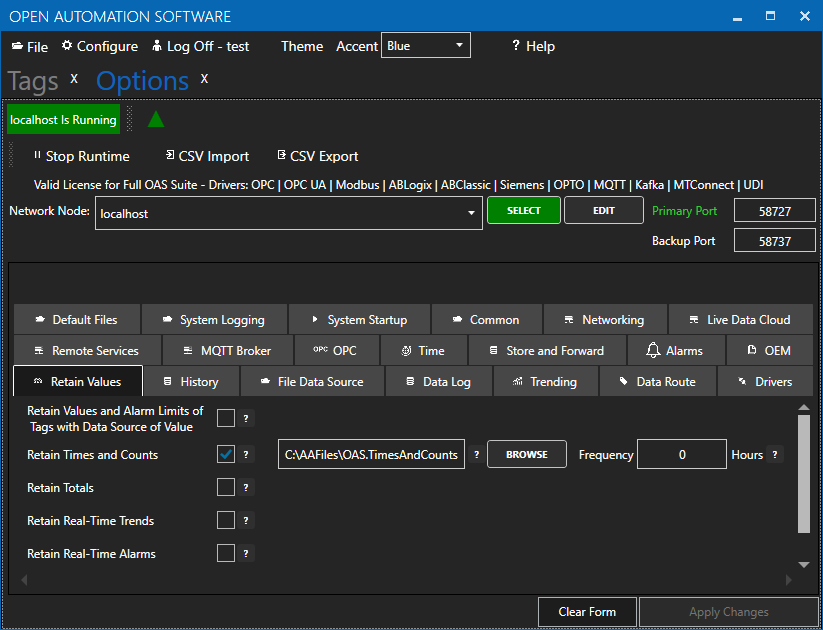# Total

View the following video for explanation of each parameter and how to enable Total for a tag:• 00:00 – Introduction
• 00:17 – Define Properties
• 00:30 – Create a New Tag
• 03:30 – Totalization Process
• 06:42 – Reset Function
• 08:34 – Control the active state
• 09:53 – Set the initial value
• 11:10 – Persist the total when system is restarted
• 12:51 – More Questions

When this feature is enabled the current value will be summed at the sample rate specified with each value multiplied by the total gain before addition to the total.

## Total Variables:

The Total feature will keep track of the following:

• Total – The summation of all sampled values.
• TotalSamples – The number of samples added since the last total reset.
• TotalAverage – The total divided by the number of samples.
• TotalPrevious – The previous total when the last reset was performed.
• TotalPreviousSamples – The previous number of samples when the last reset was performed.
• TotalAverage – The previous average when the last reset was performed.

The following image shows how the points will appear for connection in a client.## Configuration Properties:The following configuration properties are used to enable and control the summation of values.

### Enable Total

Enables the summation of the current value to update the Total variables.

### Total Active

When enabled totalizing is active at the specified sample rate.
When disabled totalizing is paused.

### Total Active by Tag

When enabled Active state is controlled by a Boolean tag value.

### Total Sample Rate

The rate at which the value * gain is added to the total in seconds.

### Total Gain

The gain will be multiplied to the value before adding to the total.
Use a negative gain or value if you want the total to decrease on each sample.

### Total Gain by Tag

When enabled the gain to multiply to value is controlled by Tag.

### Total Initial Value

The value that the total will be initialized to when the total is reset.

### Total Reset Daily

When enabled the total is set to the Total Initial Value on the reset hour and minute.

### Total Reset Monthly

When enabled the total is set to the Total Initial Value on the reset hour and minute on the first day of the month.

### Total Reset by Tag

When enabled a Boolean tag transition from false to true will set the total to the Total Initial Value.

## Retain Totals on Service Restart

To retain the total values after a system restart go to Configure-Options-Retain Values and enable to retain Totals to a file.You can optionally specify a save frequency in hours to save the live total values at a specified rate.  If the save Frequency is left at 0 the totals are only saved when the runtime is stopped or an orderly system shutdown.